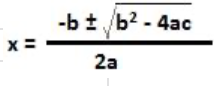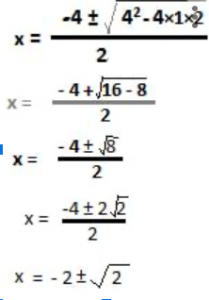What is the quadratic formula? It is an alternative to solving a quadratic equation. What does it mean to solve a quadratic equation? That means to find roots of a quadratic equation. Roots of a quadratic equation are values of the variable in the quadratic equation, say x, which reduce the value of the equation to zero (0).

Now consider a quadratic equation of the standard form:

ax2 + bx + c = 0

We know a quadratic equation can be solved by factoring method.But, not all trinomials in the form of a quadratic equation can be solved by the factoring method.

Consider for example the following equation:

x2 + 4x + 2 = 0

In this quadratic equation, the product of roots, which have yet to be solved for, is 1 × 2, i.e. 2.

But what two integers can be there, having a product of 2, with a sum -4 and ax2 + bx + c = 0. None, indeed !

Therefore, the need for an alternative for finding the roots of this quadratic equation arises.

The alternative is called the “The Quadratic Formula”

When factoring a quadratic equation gets difficult, then the quadratic formula enables us to find roots of a quadratic equation of the form ax2 + bx + c = 0In this formula, x is roots of the quadratic equation ax2 + bx + c = 0

Consider the quadratic equation ax2 + bx + c = 0

Now, ax2 + bx = – c

Divide both sides of the equation by a. So,

x2 +( b/a) x =-c/a

let us add b2/4a2 to both sides of above, so we get

x2 +( b/a) x+ b2/4a2 = -c + (b2/4a2 )

factorize the perfect square trinomial in the left side, and apply LCM in the right

(x + (b/2a))2 = (b2 – 4ac)/4a2

Take square roots on both sides,

x + (b/2a) = ± [√(b2 – 4ac)/2a]

Finally,This is the solution to the general quadratic equation ax2 + bx + c

And the solution## Example 1:

Solve the quadratic equation x2 + 4x + 2 = 0

Solution :

As tried above, this quadratic equation cannot be solved by the factoring method.

Therefore, let us use the quadratic formula derived above.

Comparing the given quadratic equation x2 + 4x + 2 = 0 with the general form ax2 + bx + c

We see, a = 1, b = 4, c = 2. Now the roots of the quadratic equation x2 + 4x + 2 = 0, using the quadratic formula areNow, square root of 2 is an irrational number, therefore factoring method could not work for

finding roots of this quadratic equation.

Therefore, the two roots of the quadratic equation x2 + 4x + 2 = 0 are

x = – 2 + √2 or x = – 2 – √2